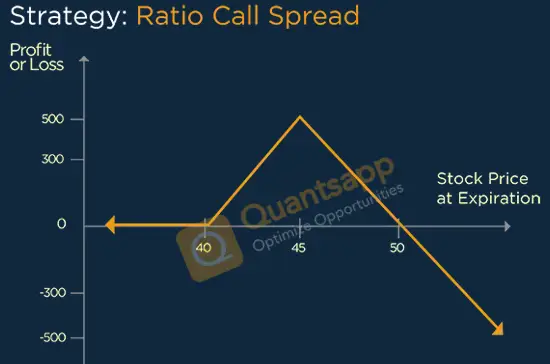# Ratio Call Spread Option Strategy# What is Ratio Call Spread Option Strategy?

• Ratio Call Spread Option Strategy is Neutral to moderately bullish Strategy. In this we expect stock to remain below upper breakeven point. Ratio Call Spread is a blend of bull call spread and a Naked call sell, where naked call will be the same as higher strike of bull call spread.

## When to Execute?

• Ratio Call Spread should be implemented when expect slow rally up to the sold strike price and expecting decline in volatility.

• In ratio call spread we generally buy one lot of At-the-money Call and sell two lots of Out-of-money Call option. The variation to ratio call spread is to use different ratios such as 1X3,2X3 or 3X5.

#### Breakeven for Ratio Call Spread

• As Net Credit = Higher Strike Price + Difference in strike + Net Premium Received. As net Debit = there will be two breaks even. Upper Breakeven = Higher Strike Price – Difference in strike + Net Premium paid. Lower Breakeven = Lower Strike + Net premium Paid.

#### What will be maximum profit?

• Maximum profit would occur at a higher strike price at expiry. Here the two short calls will expire worthless and long calls will turn In-the-money (ITM). It can be calculated as follows = Higher Strike – Lower Strike + net premium received.

#### What will be maximum loss?

• Maximum loss is undefined above the higher breakeven because this is not a complete hedge strategy as we short on more calls than buying.